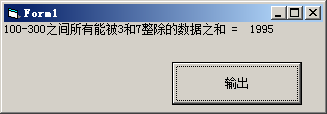# VB编程：计算100-300之间所有能被3和7整除的数据之和 （图）

Private Sub Command1_Click()
Dim sum As Integer
sum = 0 '设初值
Dim i As Integer
For i = 100 To 300
If 0 = i Mod 3 And 0 = i Mod 7 Then sum = sum + i ' 能被3整除并且被7整除,则累加
Next i
Print "100-300之间所有能被3和7整除的数据之和 = "; (sum) '循环结果就输出结果
End Sub<< 上一篇 下一篇 >>